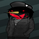1538浏览
Backtesting.

" Laguerre RSI is based on John EHLERS' Laguerre Filter to avoid the noise of RSI .

Change alpha coefficient to increase/decrease lag and smoothness.

Buy when Laguerre RSI crosses upwards above 20.
Sell when Laguerre RSI crosses down below 80.

While indicator runs flat above 80 level, it means that an uptrend is strong.
While indicator runs flat below 20 level, it means that a downtrend is strong. "

Developer: John EHLERS
Author: KivancOzbilgic

## 评论Hello mertriver1, thank you !
Do you know how to convert this strategy into an automatic alert?Thomas_MND
@Thomas_MND, You are welcome! Click on the link below for the original script. Set plots level 50 to create an alarm that fits my strategy.mertriver1
@mertriver1, I dont know how to do it can you copy me the code please ?Thomas_MND
@Thomas_MND,

// This source code is subject to the terms of the Mozilla Public License 2.0 at https://mozilla.org/MPL/2.0/
// Developer: John EHLERS
//@version=3
// Author:Kıvanç Özbilgiç
strategy("Laguerre RSI", shorttitle="LaRSI", overlay=false)
src = input(title="Source", defval=close)
alpha = input(title="Alpha", type=float, minval=0, maxval=1, step=0.1, defval=0.2)
colorchange = input(title="Change Color ?", type=bool, defval=false)

Date1 = input(true, title = "=== Date Backtesting ===")
FromDay1 = input(defval = 1, title = "From Day", minval = 1, maxval = 31)
FromMonth1 = input(defval = 1, title = "From Month", minval = 1, maxval = 12)
FromYear1 = input(defval = 2020, title = "From Year", minval = 2017)

ToDay1 = input(defval = 1, title = "To Day", minval = 1, maxval = 31)
ToMonth1 = input(defval = 1, title = "To Month", minval = 1, maxval = 12)
ToYear1 = input(defval = 9999, title = "To Year", minval = 2017)

start1 = timestamp(FromYear1, FromMonth1, FromDay1, 00, 00)
finish1 = timestamp(ToYear1, ToMonth1, ToDay1, 23, 59)
window1() => time >= start1 and time <= finish1 ? true : false

gamma=1-alpha
L0 = 0.0
L0 := (1-gamma) * src + gamma * nz(L0)
L1 = 0.0
L1 := -gamma * L0 + nz(L0) + gamma * nz(L1)

L2 = 0.0
L2 := -gamma * L1 + nz(L1) + gamma * nz(L2)

L3 = 0.0
L3 := -gamma * L2 + nz(L2) + gamma * nz(L3)

cu= (L0>L1 ? L0-L1 : 0) + (L1>L2 ? L1-L2 : 0) + (L2>L3 ? L2-L3 : 0)

cd= (L0<L1 ? L1-L0 : 0) + (L1<L2 ? L2-L1 : 0) + (L2<L3 ? L3-L2 : 0)

temp= cu+cd==0 ? -1 : cu+cd
LaRSI=temp==-1 ? 0 : cu/temp

Color = colorchange ? (LaRSI > LaRSI ? green : red) : blue
plot(100*LaRSI, title="LaRSI", linewidth=2, color=Color, transp=0)
plot(20,linewidth=1, color=maroon, transp=0)
plot(80,linewidth=1, color=maroon, transp=0)

strategy.entry("Long", true, when = window1() and crossover(cu, cd))
strategy.entry("Short", false, when = window1() and crossunder(cu, cd))